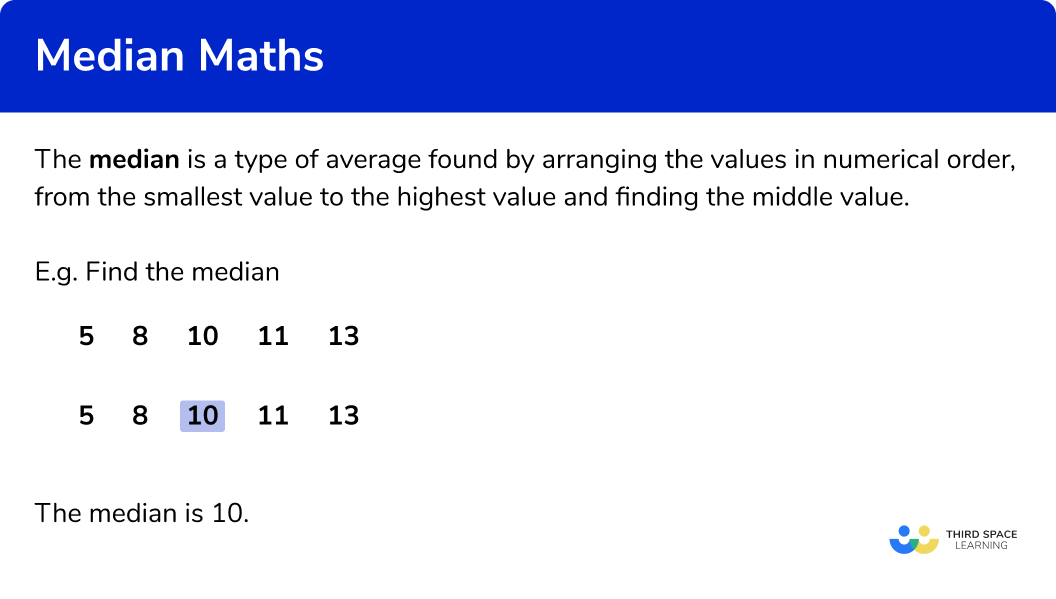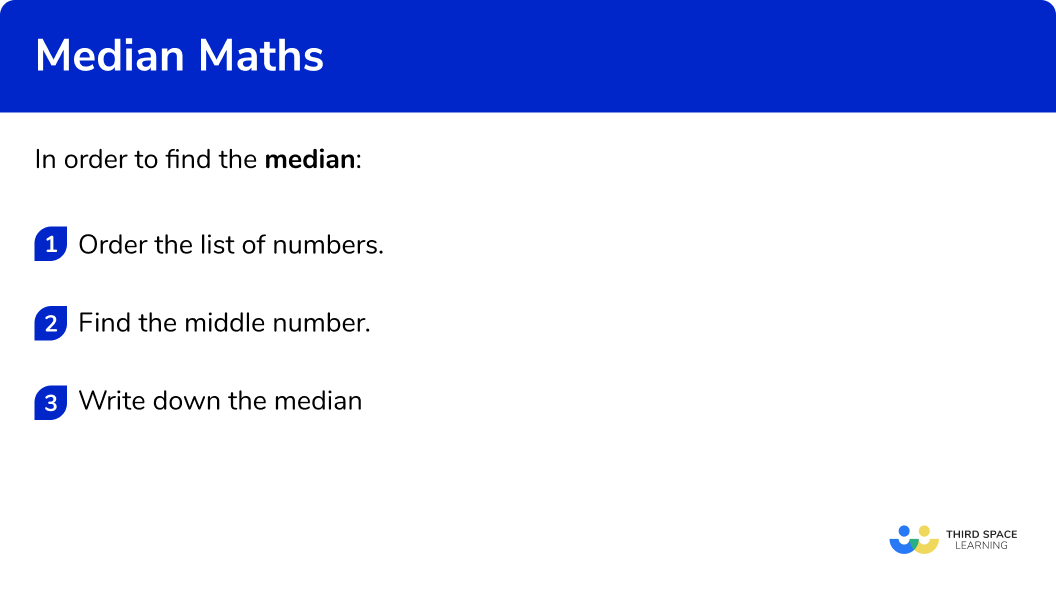# Median

Here we will learn about the median, including what the median is and how to find it.

There are also median worksheets based on Edexcel, AQA and OCR exam questions, along with further guidance on where to go next if you’re still stuck.

## What is the median?

The median is a type of average found by arranging the values in numerical order, from the smallest value to the highest value and finding the middle value.

E.g. Find the median

The median is 10

When there is an odd number of values, finding the middle value can be relatively straightforward.

We can use a formula to help us find the position of the median:

\text{median}=(\frac{n+1}{2})^\text{th} \ \text{value}

Where n is the number of values. So when n=5 , the median is the 3 rd value.

\text{position of the median} = \text{median}=(\frac{n+1}{2})^\text{th} \ \text{value}=(\frac{5+1}{2})^\text{th}=3^\text{rd} \ \text{value}

However when there is an even number of values, we get a middle pair. Here, to find the median we need to find the midpoint of the middle pair of values. We can do this by sight or by finding the mean of the two middle numbers. We can add them together and then half the total.

E.g. Find the median

The middle pair of values is 8 and 10 .  The midpoint of 8 and 10 is 9 .

We find this by adding the two values together and dividing by 2 .

\frac{8+10}{2}=\frac{18}{2}=9

The median is 9

We can use a formula to help us find the position of the median:

\text{median}=(\frac{n+1}{2})^\text{th} \ \text{value}

Where n is the number of values. So when n=6 , the median is the 3.5 th value, so the midpoint of the 3 rd and 4 th values.

\text{position of the median} = \text{median}=(\frac{n+1}{2})^\text{th} \ \text{value}=(\frac{6+1}{2})^\text{th}=3.5^\text{rd} \ \text{value}

The median is a measure of central tendency because it describes a set of numbers by identifying a central position within the data.

### What is the median?## How to find the median

In order to find the median:

1. Order the list of numbers.
2. Find the middle number.
3. Write down the median.

### How to find the median## Related lessons on mean, median, mode

Median is part of our series of lessons to support revision on mean, median, mode. You may find it helpful to start with the main mean, median, mode lesson for a summary of what to expect, or use the step by step guides below for further detail on individual topics. Other lessons in this series include:

## Median maths examples

### Example 1: odd number of values

Find the median of this set of data:

1. Order the list of numbers.

The list of numbers is not in order, so we need to put the numbers in order from smallest number to largest number.

2Find the middle number.

To find the middle number we can count in from the bottom and the top of the list.

3Write down the median.

The median value is 11

Alternatively there is a formula you can use to find the position of the median.

\text{median}=(\frac{n+1}{2})^\text{th} \ \text{value}

Where n is the number of values. So when n=5 , the median is the 3 rd value.

\text{median}=(\frac{n+1}{2})^\text{th} \ \text{value}=(\frac{5+1}{2})^\text{th}=3^\text{rd} \ \text{value}

The median is 11

### Example 2: odd number of values

Find the median of the following data:

The list of numbers is not in order, so we need to put the numbers in order from smallest to largest value.

To find the middle number we can count in from the bottom and the top of the list.

The median is 10

Alternatively there is a formula you can use to find the position of the median.

\text{median}=(\frac{n+1}{2})^\text{th} \ \text{value}

Where n is the number of values. So when n=7 , the median is the 4 th value.

\text{position of the median} = \text{median}=(\frac{n+1}{2})^\text{th} \ \text{value}=(\frac{7+1}{2})^\text{th}=4^\text{th} \ \text{value}

The median is 10

### Example 3: odd number of values

Find the median:

The list of numbers is not in order, so we need to put the numbers in order from smallest to largest value.

To find the middle number we can count in from the bottom and the top of the list.

The median is 9

Alternatively there is a formula you can use to find the position of the median.

\text{median}=(\frac{n+1}{2})^\text{th} \ \text{value}

Where n is the number of values. So when n=9 , the median is the 5 th value.

\text {position of the median} = \text{median}=(\frac{n+1}{2})^\text{th} \ \text{value}=(\frac{9+1}{2})^\text{th}=5^\text{th} \ \text{value}

The median is 9

### Example 4: even number of values

Find the median:

The list of numbers is not in order, so we need to put the numbers in order from smallest to largest value.

To find the middle number we can count in from the bottom and the top of the list.

Since there is an even number of values we have a middle pair. We need to find the mean of this middle pair.

\frac{4+6}{2}=\frac{10}{2}=5

The median is 5

Alternatively there is a formula you can use to find the position of the median.

\text{median}=(\frac{n+1}{2})^\text{th} \ \text{value}

Where n is the number of values. So when n=6 , the median is the 3.5 th value. This would be the average of the 3 rd and 4 th values.

\text {position of the median} = \text{median}=(\frac{n+1}{2})^\text{th} \ \text{value}=(\frac{6+1}{2})^\text{th}=3.5^\text{th} \ \text{value}

### Example 5: even number of values

Find the median:

The list of numbers is not in order, so we need to put the numbers in order from smallest to largest value.

To find the middle number we can count in from the bottom and the top of the list.

Since there is an even number of values we have a middle pair. We need to find the mean of this middle pair.

\frac{16+17}{2}=\frac{33}{2}=16.5

The median is 16.5

Alternatively there is a formula you can use to find the position of the median.

\text{median}=(\frac{n+1}{2})^\text{th} \ \text{value}

Where n is the number of values. So when n=8 , the median is the 4.5 th value. This would be the average of the 4 th and 5 th values.

\text{position of the median} = \text{median}=(\frac{n+1}{2})^\text{th} \ \text{value}=(\frac{8+1}{2})^\text{th}=4.5^\text{th} \ \text{value}

The median is 16.5

### Example 6: even number of values

Find the median:

The list of numbers is not in order, so we need to put the numbers in order from smallest to largest value.

To find the middle number we can count in from the bottom and the top of the list.

Since there is an even number of values we have a middle pair. We need to find the mean of this middle pair.

\frac{8+11}{2}=\frac{19}{2}=9.5

The median is 9.5

Alternatively there is a formula you can use to find the position of the median.

\text{median}=(\frac{n+1}{2})^\text{th} \ \text{value}

Where n is the number of values. So when n=10 , the median is the 5.5 th value. This would be the average of the 5 th and 6 th values.

\text{position of the median} = \text{median}=(\frac{n+1}{2})^\text{th} \ \text{value}=(\frac{10+1}{2})^\text{th}=5.5^\text{th} \ \text{value}

The median is 9.5

### Common misconceptions

• Check which average you are being asked for

Check if you have been asked for the median, mode or mean average.

• Numbers in ascending order

Check that the list of numbers is in numerical order before finding the median number.

• The median can be a number that is not in the original data set

When there is an odd number of values, the median is one of the original values.

When there is an even number of values, it is acceptable that the median is NOT one of the original values.

### Practice median maths questions

1. Find the median1314.51514Put the numbers in order from the smallest to largest value. Then find the middle value.The middle value is 15 , so this is the median.

2. Find the median17141115Put the numbers in order from the smallest to largest value. Then find the middle value.The middle value is 14 , so this is the median.

3. Find the median13171518Put the numbers in order from the smallest to largest value. Then find the middle value.The middle value is 18 , so this is the median.

4. Find the median202321.521Put the numbers in order from the smallest to largest value. Then find the middle value.The middle pairs of values are 20 and 23 . The mean of these is 21.5 . The median is 21.5 .

\frac{20+23}{2}=\frac{43}{2}=21.5

5. Find the median11159.514Put the numbers in order from the smallest to largest value. Then find the middle value.The middle pairs of values are 13 and 15 . The mean of these is 14 . The median is 14 .

\frac{13+15}{2}=\frac{28}{2}=14

6. Find the median15.519.51813Put the numbers in order from the smallest to largest value. Then find the middle value.The middle pairs of values are 13 and 18 . The mean of these is 14 . The median is 15.5 .

\frac{13+18}{2}=\frac{31}{2}=15.5

### Median maths GCSE questions

1. Here is a list of numbers.

4 8 3 12 6

Work out the median.

(1 mark)

6

(1)

2. Here is a list of numbers.

23 17 11 21 19 18 13

Work out the median.

(2 marks)

11 13 17 18 19 21 23
For putting the numbers in order

(1)

18

(1)

3. These are the heights, in metres, of members of a water polo team.

1.71 1.78 1.67 1.72 1.83 1.79 1.76

Find the median height of the 7 players.

(2 marks)

1.67 1.71 1.72 1.76 1.78 1.79 1.83
For putting the numbers in order

(1)

1.76

(1)

## Learning checklist

You have now learned how to:

• Find the median when there is an odd number of values
• Find the median when there is an even number of values

## Still stuck?

Prepare your KS4 students for maths GCSEs success with Third Space Learning. Weekly online one to one GCSE maths revision lessons delivered by expert maths tutors.

Find out more about our GCSE maths tuition programme.